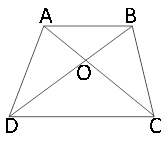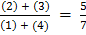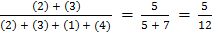### Sample Problem

The area of the following trapezoid is 24 cm2. If 5 times its longer base is equal to 7 times its shorter base, then what is the difference between the area of ∆DOC and the area of∆ AOB?

cm2#### SolutionWe label the sections as shown. Since 7AB = 5CD, we have.

Since the height of ABC and ACD are the same, the ratio of their areas is the ratio of their bases, so.

Thus, the ratio of ABC to ABCD is,

so its area is 24 × 5/12 = 10 cm2. ADC = ABCD-ABC, so ADC = 24 - 10 = 14 cm2. We see that ADC and BDC have the same area because they have the same base and height. Thus,